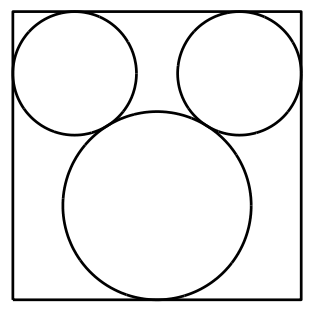# Reminds Me of Mickey Mouse

Geometry Level 3Three circles fit inside a square as shown where two small circles touch a large circle. Given that the radius of the small circles is 3 and the side length of the square is 14, find the radius of the larger circle to one decimal place.

×